Share

# Concept of Solid Shapes

Course

#### description

• Right Circular Cylinders, Cuboid, Cylinder, Cone, Cube, Pyramid

#### notes

The objects around us come in various different shapes and sizes. We can see triangles, squares, and circles everywhere. Some of these shapes like the shape of a house have length, breadth, and height and are the 3D or the three-dimensional objects. Others like a sheet of paper can be imagined to have a length and a breadth only. Such objects are the two-dimensional objects and form the 2D shapes.

(i) 2D Figures:
A shape or a figure that has a length and a breadth only is a 2D shape. The sides are made of straight or curved lines. They can have any number of sides. Plane figures made of lines are called polygons. Triangle and squares are examples of polygons. We will classify the figures we have seen in terms of what is known as the dimension. A plane object that has a length and a breadth only have 2 Dimensions.

Examples of 2D shapes and figures
We say that a figure that is drawn on paper and has only length and breadth is a 2-d figure.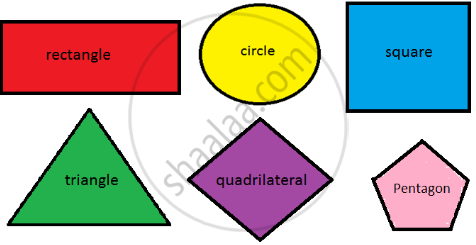(ii) 3D Shapes :
In our day to day life, we see several objects like books, ball, ice-cream cone etc, around us which have different shapes. One thing common to most of these objects is that they all have some length, breadth and height or depth. Therefore they all occupy space and have three dimensions.

Examples of 3D shapes and figures
We can say that the figures which we draw on paper and have length, breadth and height are called 3-d figures. Hence a 3D shape has three dimensions. The D in 3D stands for dimensional.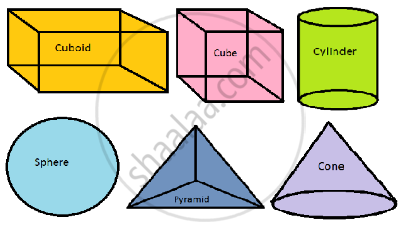Polyhedron :
A solid shape bounded by polygons is called a polyhedron. The word polyhedral is the plural of word polyhedron.

⦁ Faces: Polygons forming a polyhedron are known as its faces.
⦁ Edges: Line segments common to intersecting faces of a polyhedron are known as its edges.
⦁ Vertices: Points of intersection of edges of a polyhedron are known as its vertices.In a polyhedron, three or more edges meet at a point to form a vertex.
Some examples of polyhedrons are the cuboid, cube, pyramid, triangular pyramid etc.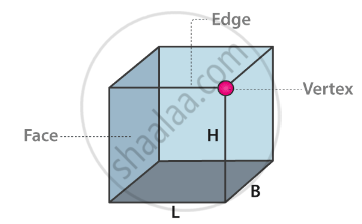The corners of the cube are its vertices. The 12 line segments that form the skeleton of the cube are its edges. The 6 flat square surfaces that are the skin of the cube are its faces.

Nets for building 3D shape :
“A net is a two-dimensional representation of a three-dimensional figure that is unfolded along its edges so that each face the figure is shown in two dimensions. In other words, a net is a pattern made when the surface of a three-dimensional figure is laid out felt showing each face of the figure.” A solid may have different nets.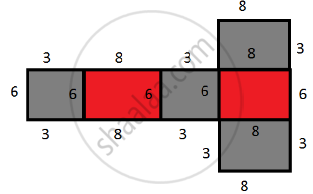You have different nets for the different shape.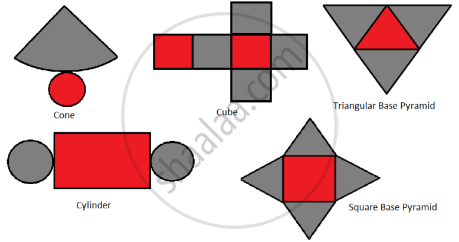Prism:
A prism is a solid, whose side faces are parallelograms and whose bases are congruent parallel rectilinear figures.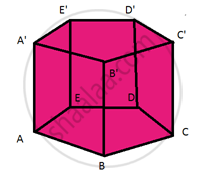If you would like to contribute notes or other learning material, please submit them using the button below.